# Resonance

(diff) ← Older revision | Latest revision (diff) | Newer revision → (diff)

The phenomenon of increasing amplitudes of forced oscillations when the frequency of the external action approximates one of the frequencies of the eigenoscillations (cf. Eigen oscillation) of a dynamical system. Resonance is simplest in a linear dynamical system. The differential equation of motion of a linear system with one degree of freedom in an environment with viscous friction and with harmonic external action takes the form:whereis a generalized coordinate,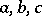are constant parameters characterizing the system, and,,are the amplitude, the frequency and the initial phase of the external action, respectively. The stationary forced oscillations occur in accordance with the harmonic law with frequencyand amplitude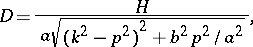where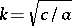is the frequency of the eigenoscillations in the absence of energy dissipation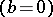. The amplitudehas a maximum value when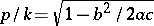, and with low energy dissipation it is close to this value when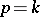. Sometimes by resonance is meant that case where. Ifthen, when, the amplitude of the forced oscillations increases proportional to time. If a linear system hasdegrees of freedom, then resonance begins when the frequency of the external force coincides with one of the eigenfrequencies of the system. With non-harmonic action, resonance may occur only when the frequencies of its harmonic spectrum coincide with the frequencies of eigenoscillations.

How to Cite This Entry:
Resonance. Encyclopedia of Mathematics. URL: http://encyclopediaofmath.org/index.php?title=Resonance&oldid=15377
This article was adapted from an original article by N.V. Butenin (originator), which appeared in Encyclopedia of Mathematics - ISBN 1402006098. See original article